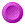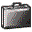# 2 0 0 9

 Regular articles:P.G. Kevrekidis, D.E. Pelinovsky, and A. Stefanov, Asymptotic stability of small solitons in the discrete nonlinear Schrodinger equation in one dimension, SIAM Journal of Mathematical Analysis 41, `2010-2030` (2009)T. Dohnal, D. Pelinovsky, and G. Schneider, Coupled-mode equations and gap solitons in a two-dimensional nonlinear elliptic problem with a separable periodic potential, Journal of Nonlinear Science, 19, `95-131` (2009)T.R.O. Melvin, A.R. Champneys, and D.E. Pelinovsky, Discrete traveling solitons in the Salerno model, SIAM Journal of Applied Dynamical Systems 8, `689–709` (2009)C. Gallo and D.E. Pelinovsky, Eigenvalues of a nonlinear ground state in the Thomas-Fermi approximation, J. Math. Anal. Appl. 355, `495–5261` (2009)R.N. Ibragimov and D.E. Pelinovsky, Incompressible viscous fluid flows in a thin spherical shell, Journal of Mathematical Fluid Mechanics 11, `60-90` (2009)M. Chugunova and D. Pelinovsky, On quadratic eigenvalue problems arising in stability of discrete vortices, Linear Algebra and its Applications 431, `962-973` (2009)Yu. Liu, D. Pelinovsky, and A. Sakovich, Wave breaking in the short-pulse equation, Dynamics of PDE 6, `291-310` (2009) Proceedings papers:M. Chugunova and D. Pelinovsky, On the uniform convergence of the Chebyshev interpolants for solitons, Mathematics and Computers in Simulations, 80, `794-803` (2009)